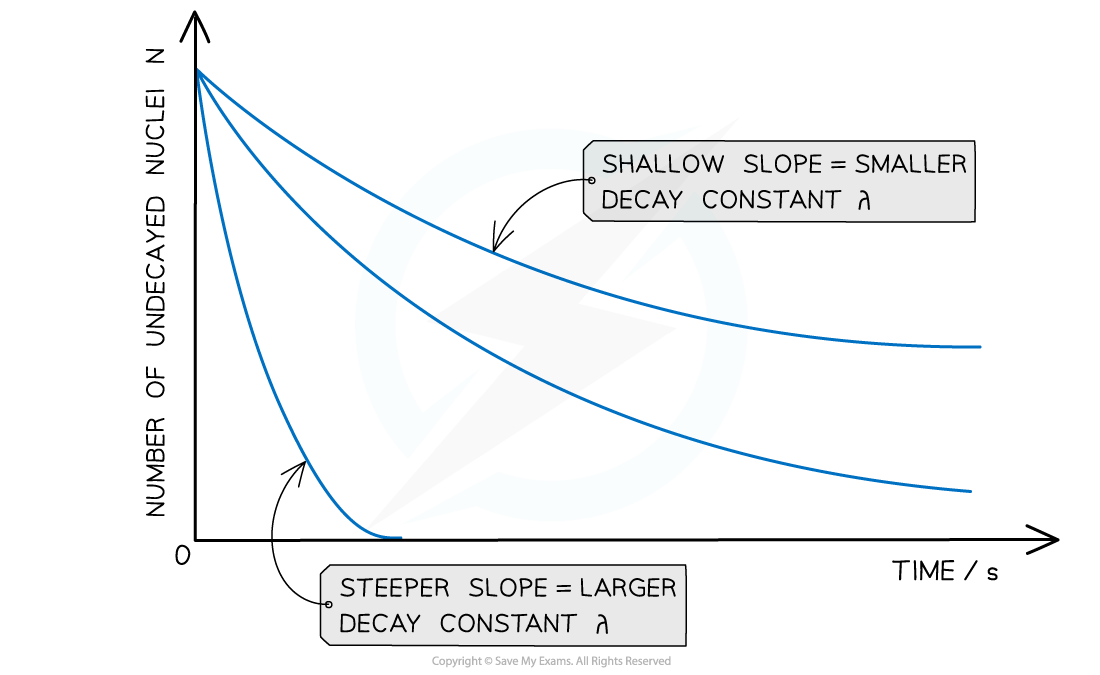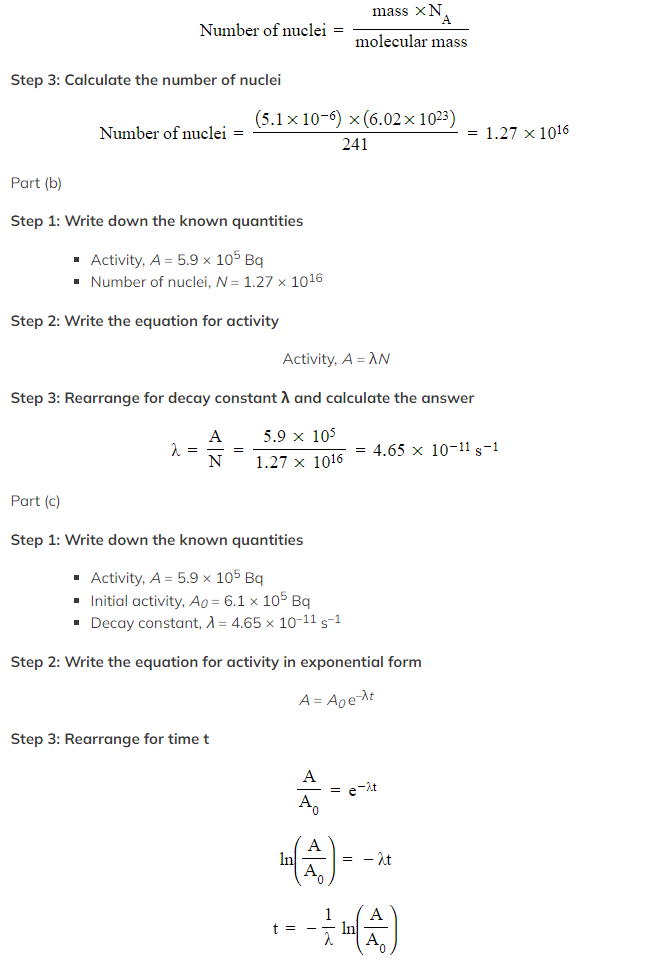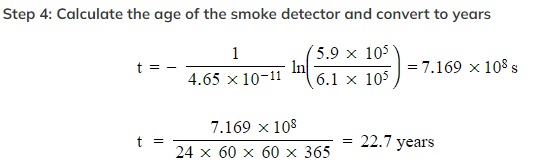# IB DP Physics: HL复习笔记12.2.6 The Law of Radioactive Decay

### The Law of Radioactive Decay

• Since radioactive decay is spontaneous and random, it is useful to consider the average number of nuclei which are expected to decay per unit of time
• This is known as the average decay rate
• As a result, each radioactive element can be assigned a decay constant
• The decay constant λ is defined as:

The probability that an individual nucleus will decay per unit of time

• When a sample is highly radioactive, this means the number of decays per unit time is very high
• This suggests it has a high level of activity
• Activity, or the number of decays per unit time can be calculated using:• Where:
• A = activity of the sample (Bq)
• ΔN = number of decayed nuclei
• Δt = time interval (s)
• λ = decay constant (s-1)
• N = number of nuclei remaining in a sample
• In radioactive decay, the number of undecayed nuclei falls very rapidly, without ever reaching zero
• Such a model is known as exponential decay
• The graph of number of undecayed nuclei against time has a very distinctive shape:Radioactive decay follows an exponential pattern. The graph shows three different isotopes each with a different rate of decay

• The key features of this graph are:
• The steeper the slope, the larger the decay constant λ (and vice versa)
• The decay curves always start on the y-axis at the initial number of undecayed nuclei (N0)
• The law of radioactive decay states:

The rate of decay of a nuclide is proportional to the amount of radioactive material remaining

• The number of undecayed nuclei N can be represented in exponential form by the equation:

N = N0 e–λt

• Where:
• N0 = the initial number of undecayed nuclei (when t = 0)
• N = number of undecayed nuclei at a certain time t
• λ = decay constant (s-1)
• t = time interval (s)
• The number of nuclei can be substituted for other quantities
• For example, the activity A is directly proportional to N, so it can also be represented in exponential form by the equation:

A = A0 e–λt

• Where:
• A = activity at a certain time t (Bq)
• A0 = initial activity (Bq)
• The received count rate C is related to the activity of the sample, hence it can also be represented in exponential form by the equation:

C = C0 e–λt

• Where:
• C = count rate at a certain time t (counts per minute or cpm)
• C0 = initial count rate (counts per minute or cpm)

#### Exam Tip

The symbol e represents the exponential constant - it is approximately equal to e = 2.718

On a calculator, it is shown by the button ex

The inverse function of ex is ln(y), known as the natural logarithmic function - this is because, if ex = y, then x = ln(y)

Make sure you are confident using the exponential and natural logarithmic functions, they are a major component of the mathematics in this topic!

### Problems Involving the Radioactive Decay Law

#### Worked Example

Strontium-90 decays with the emission of a β-particle to form Yttrium-90. The decay constant of Strontium-90 is 0.025 year -1.

Determine the activity A of the sample after 5.0 years, expressing the answer as a fraction of the initial activity A0.

Step 1: Write out the known quantities

• Decay constant, λ = 0.025 year -1
• Time interval, t = 5.0 years
• Both quantities have the same unit, so there is no need for conversion

Step 2: Write the equation for activity in exponential form

A = A0 e–λt

Step 3: Rearrange the equation for the ratio between A and A0Step 4: Calculate the ratio A/A0• Therefore, the activity of Strontium-90 decreases by a factor of 0.88, or 12%, after 5 years

#### Worked Example

Americium-241 is an artificially produced radioactive element that emits α-particles.

In a smoke detector, a sample of americium-241 of mass 5.1 µg is found to have an activity of 5.9 × 105 Bq. The supplier’s website says the americium-241 in their smoke detectors initially has an activity level of 6.1 × 105 Bq.

(a)Determine the number of nuclei in the sample of americium-241.
(b)Determine the decay constant of americium-241.
(c)Determine the age of the smoke detector in years.

Part (a)

Step 1: Write down the known quantities

• Mass = 5.1 μg = 5.1 × 10-6 g
• Molecular mass of americium = 241
• NA = the Avogadro constant

Step 2: Write down the equation relating to the number of nuclei, mass and molecular massTherefore, the smoke detector is 22.7 years old